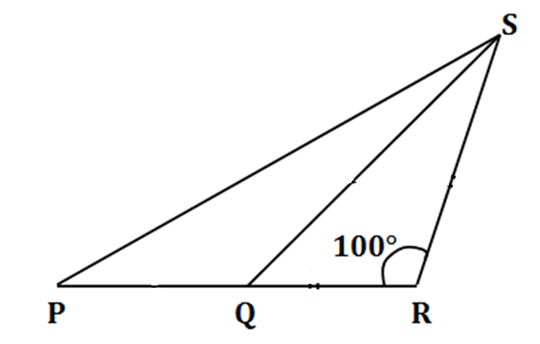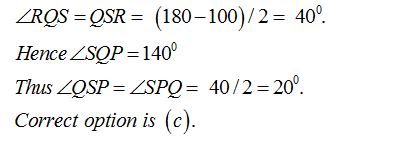• This is an assessment test.
• These tests focus on geometry and mensuration and are meant to indicate your preparation level for the subject.
• Kindly take the tests in this series with a pre-defined schedule.

## Geometry and Mensuration: Test 23

Congratulations - you have completed Geometry and Mensuration: Test 23. You scored %%SCORE%% out of %%TOTAL%%. You correct answer percentage: %%PERCENTAGE%% . Your performance has been rated as %%RATING%%
 Question 1
If the medians of two equilateral triangles are in the ratio 1:2 then what is the ratio of their sides?
 A 1: 2 B 2: 3 C 3: 2 D √3: √2
Question 1 Explanation:
$\displaystyle \begin{array}{l}Median\text{ }of\text{ }an\text{ }equilateral\text{ }triangle=\frac{\sqrt{3}}{2}a\\By\text{ }given\text{ }condition\\\frac{\frac{\sqrt{3}}{2}{{a}_{1}}}{\frac{\sqrt{3}}{2}{{a}_{2}}}=\frac{1}{2}\\\therefore \,\,\frac{{{a}_{1}}}{{{a}_{2}}}=\frac{1}{2}\end{array}$
 Question 2
The hypotenuse of a right triangle is 3√10 unit. If the smaller side is tripled and the longer side is doubled, new hypotenuse becomes 9√5 unit. What are the lengths of the smaller and longer sides of the right triangle respectively?
 A 5 unit and 9 units B 5 units and 6 units C 3 units and 9 units D 3 units and 6 units
Question 2 Explanation:
Let the sides be x , y where x<y
x2 + y2 =(3√10)2 = 90
9x2 + 4y2 =(9√5)2 = 405
Solving the two equations
x = 3 and y=9
 Question 3
In the figure given above, PQ = QS and QR = RS. If ∠SRQ = 100°, how many degrees is ∠QPS?A 40° B 30° C 20° D 15°
Question 3 Explanation:Question 4
ABC is a right angled triangle, right angled at C and p is the length of the perpendicular from C on AB. If a, b and c are the lengths of the sides BC, AC and AB respectively, then which one of the following is correct?
 A (a2 + b2)p2 = a2b2 B a2 + b2 = a2b2p2 C p2= a2 + b2 D p2= a2 –b2
Question 4 Explanation:$\begin{array}{l}\frac{1}{2}pc=\frac{1}{2}ab\,\,\left( Since\text{ }area\text{ }of\text{ }same\text{ }triangles \right)\\{{p}^{2}}{{c}^{2}}={{a}^{2}}{{b}^{2}}\\=>{{p}^{2}}({{a}^{2}}+{{b}^{2}})={{a}^{2}}{{b}^{2}}\end{array}$
 Question 5
AB, EF and CD are parallel lines. Given that, EF= 5 cm GC = 10 cm, AB = 15 cm and DC = 18 cm. What is the value of AC?A 20 cm B 24 cm C 25 cm D 28 cm
Question 5 Explanation:$\begin{array}{l}\because AB||EF||CD\\\frac{EF}{CD}=\frac{EG}{GC}\\=>\frac{EF}{18}=\frac{5}{10}\\=>EF=9\\In\vartriangle ABC\text{ }and\vartriangle EFC\text{ }\left( similar\text{ }triangles \right),\\\frac{EF}{AB}=\frac{EC}{AC}\\=>9/15=15/AC\\AC=(15\times 15)/9=25cm\end{array}$
Once you are finished, click the button below. Any items you have not completed will be marked incorrect.
There are 5 questions to complete.
 ← List →